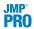• simple and multiple linear regression
 • analysis of variance and covariance
 • random effect, nested effect, mixed effect, repeated measures, and split plot models
 • nominal and ordinal logistic regression
 • multivariate analysis of variance (MANOVA)
 • canonical correlation and discriminant analysis
 • loglinear variance (to model the mean and the variance)
 • generalized linear models (GLM)
 • parametric survival and proportional hazards
 • response screening, for studying a large number of responses
 •mixed models with a range of covariance structures
 • generalized regression models including the elastic net, lasso, and ridge regression
 • partial least squares
Contents